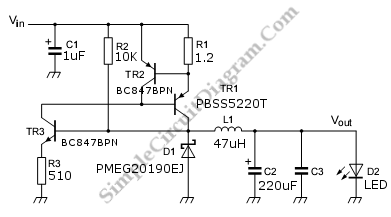# Discrete High Current Switch Mode LED Driver

This is a discrete high current switch mode LED driver circuit. The basics of this circuit is a buck-converter principle. The efficiency of this circuit is 80 to 90 %. This circuit can be applied on automotive lighting applications. The advantages of this circuit are low component and low cost. Here is the circuit:The voltage input range of this circuit is +6V to +8V. This circuit uses 47uH inductor L1 that determine the max frequency < 100Khz and the efficciency is about 80%. The smaller the inductor, the higher the maximum switching frequency of the circuit. Using the component on the figure, the current output is 300mA. This ciruit uses a Schottky diode to provide a current path for the LED current during t(off). [Source: NXP Semiconductors Application Note]